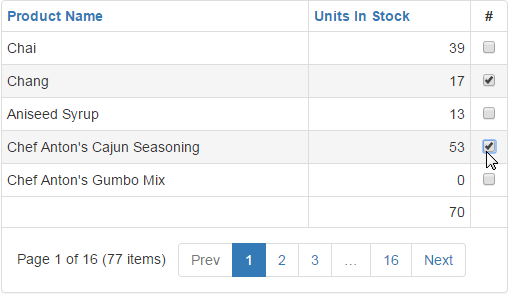21.2 (EAP/Beta)
21.1
20.2
20.1
19.2
19.1
18.2
18.1
17.2

# Create Custom Summaries

Total summaries and group summaries provide five predefined aggregate functions. These functions allow you to calculate the number of rows, the maximum and minimum values, the sum and the average value. If you need to calculate a summary value using an aggregate function not included in the predefined set, set the summary item’s ASPxSummaryItemBase.SummaryType property to Custom and handle the Grid View control’s ASPxGridBase.CustomSummaryCalculate event.

The ASPxGridBase.CustomSummaryCalculate event fires for each row involved in summary calculation. Take into account the difference between calculating the total and group summary values:

• When calculating a total summary value, the event is raised for each data row in the grid.
• When calculating a group summary value, the event fires for each data row within the currently processed group.

Additionally, the event is raised before and after processing rows, which can be used to perform any initialization and finalization.

## Example

This example shows how to implement a custom summary calculation.

1. Create a summary item within the ASPxGridView.TotalSummary collection, and customize its settings, as shown below:

``````<TotalSummary>
<dx:ASPxSummaryItem
FieldName="UnitsInStock"
ShowInColumn="Units In Stock"
SummaryType="Custom" />
</TotalSummary>
``````
2. Handle the ASPxGridBase.CustomSummaryCalculate event to perform custom calculations in the code-behind. This example demonstrates how to calculate a summary only for selected rows.

Note that in order to process selection changes on the server side, you can either set the ASPxGridBehaviorSettings.ProcessSelectionChangedOnServer property to true or handle the ASPxClientGridView.SelectionChanged client event, and call the ASPxClientGridView.PerformCallback client method.

The image below shows the result:``````int totalSum;
protected void grid_CustomSummaryCalculate(object sender, DevExpress.Data.CustomSummaryEventArgs e) {
// Initialization.
if (e.SummaryProcess == DevExpress.Data.CustomSummaryProcess.Start)
totalSum = 0;
// Calculation.
if (e.SummaryProcess == DevExpress.Data.CustomSummaryProcess.Calculate)
if (grid.Selection.IsRowSelectedByKey(e.GetValue(grid.KeyFieldName)))
totalSum += Convert.ToInt32(e.FieldValue);
// Finalization.
if (e.SummaryProcess == DevExpress.Data.CustomSummaryProcess.Finalize)
e.TotalValue = totalSum;
}
``````